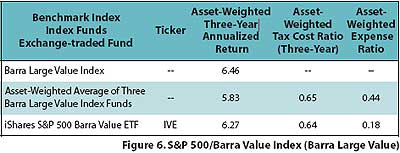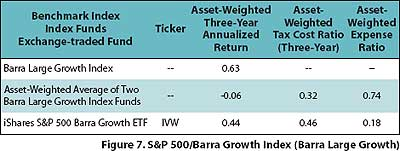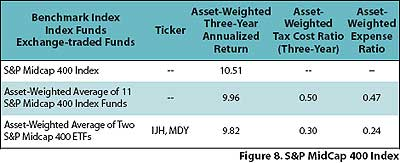# A Brave New World

July 01, 2005

S&P 500/Barra Value Index

For the S&P 500/Barra Value Index, we compared a single iShares ETF against the average of three index funds (Figure 6). The average index fund performance demonstrated a negligible advantage in tax cost ratio, but significantly worse raw performance in comparison to the ETF.The ETFs had a 44 basis point advantage in three-year annualized return, posting returns of 6.27 percent compared to 5.83 percent for the funds. The ETF return was 19 basis points lower than the raw index return, which with an average expense ratio of 18 basis points, represents virtually no tracking error. By contrast, the index fund average return was 63 basis points lower than the raw index, of which 44 basis points is accounted for by the expense ratio. Thus, the average tracking error of these three index funds was 19 basis points.

As shown in Figure 7, the two index funds that tracked the S&P/Barra Large Growth Index demonstrated superior tax efficiency compared with the iShares Barra Growth ETF. However, the dramatically larger average expense ratio among the index funds negates their tax efficiency advantage. The ETF had a positive return of 0.44 percent, 50 basis points higher than the -0.06 percent asset-weighted return for the index funds. The ETF return was within 19 basis points of the raw index, which is essentially accounted for by its expense ratio of 18 basis points. The average return of the index funds was lower than the raw index return by 69 basis points, or five basis points better than expected given an average expense ratio of 74 basis points.S&P MidCap 400 Index

Among trackers of the S&P MidCap 400 Index, two ETFs outperformed 11 index funds in the area of tax efficiency (Figure 8). In terms of performance, the index funds had the advantage by 14 basis points. This is surprising in light of the fact that the asset-weighted average expense ratio of the index funds was 23 basis points higher than the expense ratio of the two ETFs.The 11 index funds demonstrated an average tracking error of eight basis points: 47 of the 55 basis points in differential can be accounted for by the expense ratio. The tracking error of the ETFs was 45 basis points.